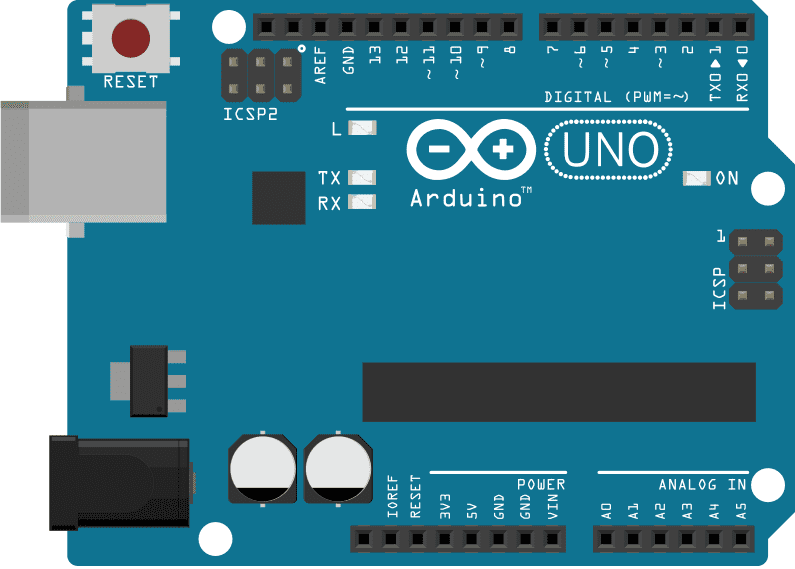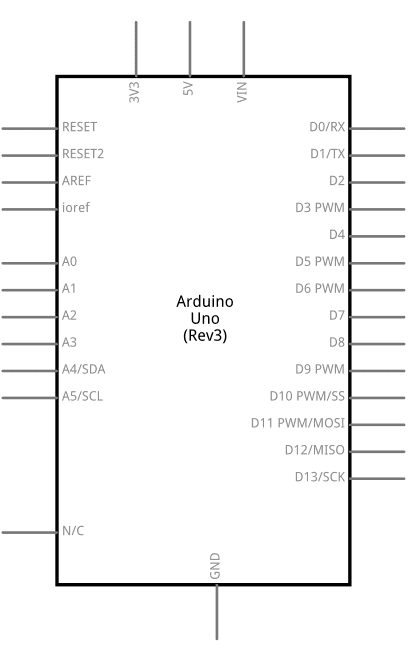### What to expect of us?### Fair pricing

#### No hidden charges.

Based upon the information you provide, You will be given an upfront estimate for your project and we'll stick to it.

## EEPROM CRC

EEPROM CRC

The microcontroller on the Arduino boards have 512 bytes of EEPROM: memory whose values are kept when the board is turned off (like a tiny hard drive).
A CRC is a simple way of checking whether data has changed or become corrupted. This example calculates a CRC value directly on the EEPROM values. This CRC is like a signature and any change in the calculated CRC means a change in the stored data. The purpose of this example is to highlight how the EEPROM object can be used just like an array.

Hardware Required
• Arduino Board
CircuitThere is no circuit for this example.

image developed using Fritzing. For more circuit examples, see the Fritzing project page

Schematicsimage developed using Fritzing. For more circuit examples, see the Fritzing project page

Code
/***

Written by Christopher Andrews.

CRC algorithm generated by pycrc, MIT licence ( https://github.com/tpircher/pycrc ).

A CRC is a simple way of checking whether data has changed or become corrupted.

This example calculates a CRC value directly on the EEPROM values.

The purpose of this example is to highlight how the EEPROM object can be used just like an array.

***/

#include <Arduino.h>
#include <EEPROM.h>

void setup() {

//Start serial

Serial.begin(9600);

while (!Serial) {

; // wait for serial port to connect. Needed for native USB port only

}

//Print length of data to run CRC on.

Serial.print("EEPROM length: ");

Serial.println(EEPROM.length());

//Print the result of calling eeprom_crc()

Serial.print("CRC32 of EEPROM data: 0x");

Serial.println(eeprom_crc(), HEX);

Serial.print("\n\nDone!");
}

void loop() {

/* Empty loop */
}

unsigned long eeprom_crc(void) {

const unsigned long crc_table = {

0x00000000, 0x1db71064, 0x3b6e20c8, 0x26d930ac,

0x76dc4190, 0x6b6b51f4, 0x4db26158, 0x5005713c,

0xedb88320, 0xf00f9344, 0xd6d6a3e8, 0xcb61b38c,

0x9b64c2b0, 0x86d3d2d4, 0xa00ae278, 0xbdbdf21c

};

unsigned long crc = ~0L;

for (int index = 0 ; index < EEPROM.length() ; ++index) {

crc = crc_table[(crc ^ EEPROM[index]) & 0x0f] ^ (crc >> 4);

crc = crc_table[(crc ^ (EEPROM[index] >> 4)) & 0x0f] ^ (crc >> 4);

crc = ~crc;

}

return crc;
}

EEPROM library reference
EEPROM Clear - Fills the content of the EEPROM memory with “0”.
EEPROM Read – Reads values stored into EEPROM and prints them on Serial.
EEPROM Write – Stores values read from A0 into EEPROM.
EEPROM Iteration – Programming examples on how to go through the EEPROM memory locations.
EEPROM Put – Put values in EEPROM using variable semantics (differs from EEPROM.write() ).
EEPROM Get – Get values from EEPROM and prints as float on serial.
EEPROM Update – Stores values read from A0 into EEPROM, writing the value only if different, to increase EEPROM life.

EXAMPLE 1: Simple CRC

unsigned char test[] = {"HELLO"};

void setup() {
// put your setup code here, to run once:
Serial.begin(19200);

}

void loop() {
// put your main code here, to run repeatedly:
Serial.println(crc32b(test),HEX);
delay(1000);

}

// ----------------------------- crc32b --------------------------------

/* This is the basic CRC-32 calculation with some optimization but no
table lookup. The the byte reversal is avoided by shifting the crc reg
right instead of left and by using a reversed 32-bit word to represent
the polynomial.
When compiled to Cyclops with GCC, this function executes in 8 + 72n
instructions, where n is the number of bytes in the input message. It
should be doable in 4 + 61n instructions.
If the inner loop is strung out (approx. 5*8 = 40 instructions),
it would take about 6 + 46n instructions. */

unsigned long crc32b(unsigned char *message) {
int i, j;

i = 0;
crc = 0xFFFFFFFF;
while (message[i] != 0) {
Byte = message[i]; // Get next byte.
Serial.println(message[i],HEX);
crc = crc ^ Byte;
for (j = 7; j >= 0; j--) { // Do eight times.
crc = (crc >> 1) ^ (0xEDB88320 & mask);
}
i = i + 1;
}
return ~crc;
}

EXAMPLE 2: BYTE ARRAY TO CRC

unsigned int CRC(byte input[], int array_size)
{
unsigned int crc16 = 0xFFFF;
int pos = 0;
int i = 0;

/*Main Code*/
for (pos = 0; pos < sizeof(input); pos++) {
crc16 ^= input[pos]; // XOR byte into least sig. byte of crc

for (i = 8; i != 0; i--) { // Loop over each bit
if ((crc16 & 0x0001) != 0) { // If the LSB is set
crc16 >>= 1; // Shift right and XOR 0xA001
crc16 ^= 0xA001;
}
else // Else LSB is not set
crc16 >>= 1; // Just shift right
}
}
/*Note, this number has low and high bytes swapped,
so swap bytes*/
return crc16;
}